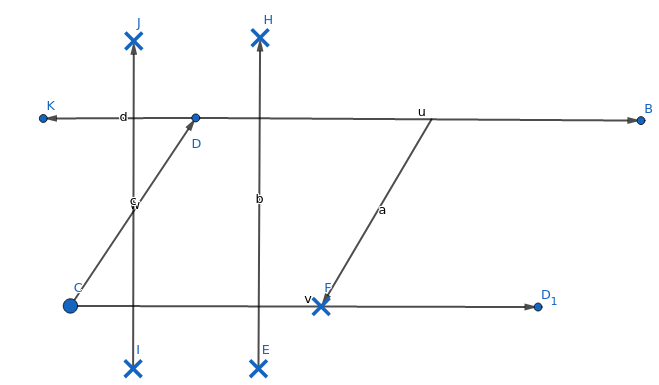QUESTION

# Construct a parallelogram ABCD, in which AB=6cm, BC=4.2cm and the height from AB=3.5cm.

Hint: We should draw the base AB upon which the given height is to be drawn. Then we may draw two perpendicular lines on the base at any two points and cut at 3.5cm on both the perpendiculars from their intersection points from the base and join them. Then we can take an arc of length 4.2cm from B and cut it on the line drawn through the perpendiculars and join B and this point of intersection to get the side BC. Now we can cut the line through the perpendiculars at 6cm from C and name it D. Finally, we can join A and D.$\therefore$ ABCD is the required parallelogram with AB=CD=6cm, BC=AD=4.2cm and a height of 3.5cm.